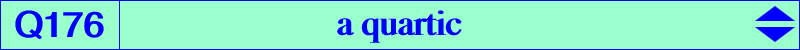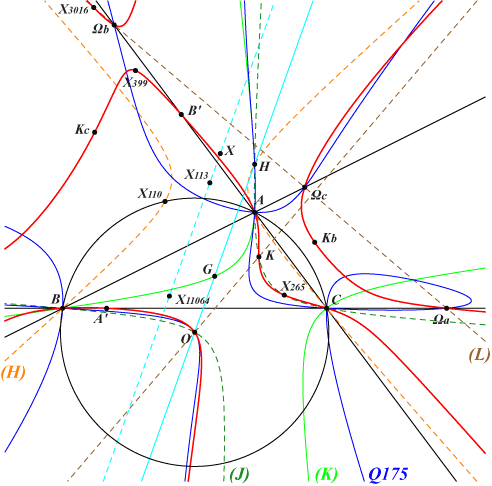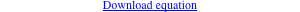too complicated to be written here. Click on the link to download a text file.X(3), X(6), X(265), X(399), X(2574), X(2575), X(3016) Ωa, Ωb, Ωc : centers of the Apollonius circles, on the Lemoine axis (L) A'B'C' : cevian triangle of X(323) KaKbKc : anticevian triangle of X(6) i.e. tangential triangle infinite points of (H), rectangular circum-hyperbola with center X(113) further details belowQ176 is the locus of points whose polar line in Q175 passes through O. Q176 has two pairs of real perpendicular asymptotes (not represented on the figure) : • two are parallel at X(11064) to those of the Jerabek hyperbola (J). • two are parallel at X to those of the circum-hyperbola (H) passing through X(4) and X(110). This is the isogonal transform of the perpendicular at O to the Euler line. X = (-2 a^4 + a^2 b^2 + b^4 + a^2 c^2 - 2 b^2 c^2 + c^4) (a^6 - 3 a^4 b^2 + 3 a^2 b^4 - b^6 - 3 a^4 c^2 - 4 a^2 b^2 c^2 + b^4 c^2 + 3 a^2 c^4 +b^2 c^4 - c^6) : : , on the lines {X5, X182}, {X30, X113}, {X146, X186}, {X156, X235}, with SEARCH = -1.72876499514970. X is the barycentric product X(30) x X(37644). Note that X and X(11064) lie on the parallel at X(113) to the Euler line. The tangents at A, B, C to Q176 concur at X(265). The tangents at Ka, Kb, Kc to Q176 concur at Kx = 4 a^6-3 a^4 b^2-b^6-3 a^4 c^2+2 a^2 b^2 c^2+b^4 c^2+b^2 c^4-c^6 : : , on the lines {X22, X69}, {X30, X146}, {X110, X858}, {X156, X1594}, with SEARCH = -3.65571049946752.   These points X and Kx are now X(46817) and X(46818) in ETC (2022-01-28).